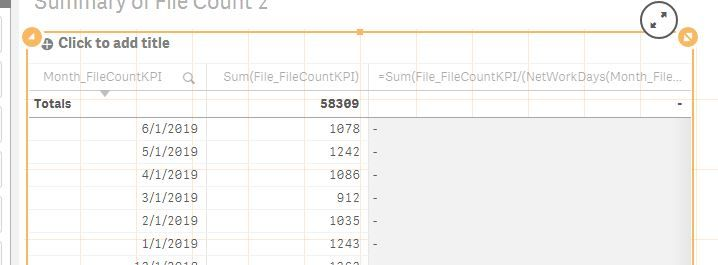# New to Qlik Sense

If you’re new to Qlik Sense, start with this Discussion Board and get up-to-speed quickly.

Announcements
Qlik Insider: SaaS capabilities for Data Integration and Analytics, May 25th! Register Now
cancel
Showing results for
Did you mean:Contributor

## Calculating the average of number occur per workdays.

Hello,

I need some help if any can help me out how to write the formula here.

So i have a simple 3 columns excel to be import into Qlik Sense.

My column 1 is the Month/Date/Year

My column 2 is sum of count for each Month/Date/Year.

I want my column 3 to do the following.

The average count of column 2 based on workdays. For example, on 6/1/2019, i have total sum of 1078 counts. In June 2019 - there is 20 working days. So it would be 1078/20 = 53.9

Then for 5/1/2019, i have 1242 counts. Working month for 5/1/2019 would be 22 workdays = 1242/22 = 56.4545

So each month will calculate what their workdays are divided by the sum of count on column 2.

How do i do this?